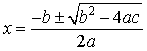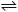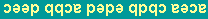CH223 - 11:00                            EXAM-2B                               Practice

 Information: gas constant:  R = 0.08206 L·atm/mole·K = 8.314 J/mole·K Avogadro's number:  NA = 6.022 ´ 1023/mol Q-dependence of free energy change:  DG  =  DGo  + RT ln(Q) normal freezing point of water:   0°C = 273K water ionization constant (25°C):  Kw = 1.00 ´ 10-14 pressure:  1 atm = 760 mmHg = 760 torr log conversion:  ln(x) = 2.303 log(x) quadratic equation:   ax2 + bx + c = 0,Multiple choice:  select one answer for each question:

1.  Calculate K for HCN(aq) + OH-(aq)CN-(aq) + H2O(l) from the reactions

 HCN(aq)H+(aq) + CN-(aq) ,   K = 4.9 ´10-10 H2O(aq)H+(aq) + OH-(aq) ,   K = 1.0 ´10-14 a) 4.9 ´ 104 b) 2.0 ´ 104 c) 4.9 ´ 10-24 d) 2.0 ´ 10-5 e) 2.0 ´ 10-25

2.  CaCO3 has Ksp= 4.8 ´10-9 .  The molar solubility of calcium carbonate in water is

 a) 4.8 ´ 10-9 M b) 7.3 ´ 10-4 M c) 6.9 ´ 10-5 M d) 7.3 ´ 10-7 M e) 6.7 ´ 10-3 M

3.   In which of the following reactions is DS° negative?

 1.   I2(g)  ®  I2(s) 2.   H2O(l)  ®  H2O(s) 3.   CO2(g)  + CaO(s) ®  CaCO3(s) a) 1 only b) 2 only c) 3 only d) 1 and 2 only e) 1, 2 and 3

4.  Find the quotient [Ag+ ]/[Ag(CN)2- ] from the complex ion formation reaction

Ag+(aq) + 2 CN-(aq)Ag(CN)2- (aq),   Kf = 3 ´1020

when the equilibrium concentration of CN- is 0.10 M.
 a) 3 ´ 10-19 b) 3 ´ 10-4 c) 3 ´ 10+2 d) 3 ´ 10-6 e) 3 ´ 10-22

5.  The pH after mixing 0.500000 mole of HCl and 0.499996 mole of NaOH in 1000 mL of water is:

 a) 5.88 b) 5.13 c) 5.40 d) 4.92 e) 5.28

6. Spontaneous chemical reactions at constant T and P always have __________.
1.  DS > 0 ,         2.   DH < 0,            3.     DG < 0

 a) 1 only b) 3 only c) 1 and 2 only d) 2 and 3 only e) 1, 2 and 3

7.   The pH of a solution that contains 4.8 mole of benzoic acid C6H5COOH (pKa = 4.20) and 3.6 mole of sodium benzoate C6H5COONa in 927 mL of water is:

 a) 3.91 b) 4.32 c) 4.15 d) 4.08 e) 4.24

8.  When DSo < 0  and DHo < 0 a chemical reaction favors

 a) products at low temperature and products at high temperature b) products at low temperature and reactants at high temperature c) reactants at low temperature and products at high temperature d) reactants at low temperature and reactants at high temperature e) none of the above

9.  Calculate the equilibrium constant for the reaction at 298K:

 3C(s) + 4H2(g)C3H8(g),      DH° = -103.8 kJ,  DS° = -269 J/K a) 1.0 ´ 1017 b) 2.1 ´ 1032 c) 1.0 d) 3.7 ´ 1019 e) 1.4 ´ 104

10.  Calculate the solubility of Cd(OH)2 (Ksp =1.2 ´10-14) in a solution buffered at pH = 7.00.

 a) 0.3 M b) 4.8 M c) 0.6 M d) 1.2 M e) 5.0 ´10-8 M

 Questions 11-15:  Titrate 72 mL of 0.200M formic acid HCOOH (Ka = 1.80 ´ 10-4) with 0.200M NaOH.

11. The pH at the starting point is

 a) 1.80 b) 3.42 c) 2.85 d) 3.16 e) 2.22

12.  The volume of NaOH needed to just reach the buffer point is:

 a) 90 mL b) 54 mL c) 120 mL d) 36 mL e) 108 mL

13.  The pH at the buffer point is

 a) 3.74 b) 4.36 c) 4.68 d) 7.00 e) 9.32

14.  At pH = 5.50  the quotient [HCOO- ] / [HCOOH] is:

 a) 36 b) 18 c) 57 d) 69 e) 180

15.  The pH at the equivalence point is

 a) 7.00 b) 8.37 c) 7.94 d) 8.62 e) 9.43

 Questions 16-20:  Titrate 84 mL of 0.125 M acid H2A (Ka1 = 3.7 ´ 10-5 , Ka2 = 8.2 ´ 10-10 ) with 0.125 M NaOH .

16. The volume of NaOH needed to just reach the first equivalence point is:

 a) 42 mL b) 84 mL c) 126 mL d) 105 mL e) 168 mL

17.  The pH at the first equivalence point is

 a) 8.27 b) 7.00 c) 4.43 d) 6.76 e) 8.14

18.  The order of concentrations at the first equivalence point is:

 a) [HA- ] > [A2- ] > [H2A] b) [H2A] = [HA- ] > [A2- ] c) [HA- ] > [H2A] > [A2- ] d) [A2- ] = [HA- ] > [H2A] e) [HA- ] > [A2- ] = [H2A]

19.  The order of concentrations at pH = 7.00 is:

 a) [HA- ] > [A2- ] = [H2A] b) [A2- ] = [HA- ] > [H2A] c) [HA- ] > [H2A] > [A2- ] d) [H2A] = [HA- ] > [A2- ] e) [HA- ] > [A2- ] > [H2A]

20.  The order of concentrations at the second equivalence point is:

 a) [HA- ] > [H2A] > [A2- ] b) [A2- ] = [HA- ] > [H2A] c) [A2- ] > [HA- ] > [H2A] d) [HA- ] > [A2- ] = [H2A] e) [H2A] = [HA- ] > [A2- ]

 KEY: